Model Files
cifar-10.zip
TensorFlow
Model
deepSea Compiled Models
cifar-10.exe
deepSea
Ubuntu

# Build a CNN on CIFAR-10 using TensorFlow¶

#### Credit: Alex Krizhevsky, University of Toronto and Vaibhav Sharma¶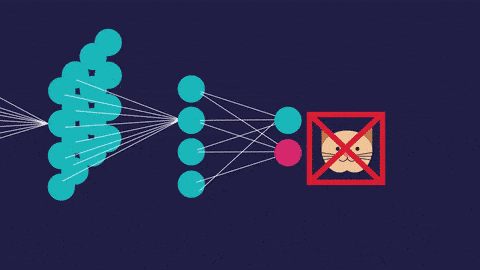Photo by Science Magazine on Fiction to Fact

## Introduction¶

The CIFAR-10 dataset is a standard dataset used in computer vision and deep learning community. It consists of 60000 32x32 colour images in 10 classes, with 6000 images per class. There are 50000 training images and 10000 test images. The mapping of all 0-9 integers to class labels is listed below.:

• 0 ~> Airplane
• 1 ~> Automobile
• 2 ~> Bird
• 3 ~> Cat
• 4 ~> Deer
• 5 ~> Dog
• 6 ~> Frog
• 7 ~> Horse
• 8 ~> Ship
• 9 ~> Truck

It is a fairly simple dataset. Hence, it provides the flexibility to play with various techniques, suh as hyperparameter tuning, regularization, training-test split, parameter search, etc. Therefore, I encourage the reader to play with this dataset after reading this tutorial.

In this tutorial, we will build a convolutional neural network model from scratch using TensorFlow, train that model and then evaluate its performance on unseen data.

## Explore CIFAR-10 dataset¶

Let us load the dataset. The dataset is split into training and testing sets. The training set consists of 50000 images, with 5000 images of each class, and the testing set consists of 10000 images, with 1000 images from each class.

In :
# Import the CIFAR-10 dataset from keras' datasets
from tensorflow.keras.datasets import cifar10

# Import this PyPlot to visualize images
import matplotlib.pyplot as plt
%matplotlib inline

import numpy as np

from sklearn.utils import shuffle

(X_train, Y_train), (X_test, Y_test) = cifar10.load_data()

In :
# Print the shapes of training and testing set
print("X_train.shape =", X_train.shape, "Y_train.shape =", Y_train.shape)
print("X_test.shape =", X_test.shape, "Y_test.shape =", Y_test.shape)

X_train.shape = (50000, 32, 32, 3) Y_train.shape = (50000, 1)
X_test.shape = (10000, 32, 32, 3) Y_test.shape = (10000, 1)


We can tell from the shapes that,

• X_train has 50000 training images, each 32 pixel wide, 32 pixel high, and 3 color channels
• X_test has 10000 testing images, each 32 pixel wide, 32 pixel high, and 3 color channels
• Y_train has 50000 labels
• Y_test has 10000 labels

Let us define constants for number of classes and its labels, to make the code more readable.

In :
NUM_CLASSES = 10
CIFAR10_CLASSES = ["airplane", "automobile", "bird", "cat", "deer",
"dog", "frog", "horse", "ship", "truck"]


Now, lets look at some random images from the training set. You can change the number of columns and rows to get more/less images.

In :
# show random images from training set
cols = 8 # Number of columns
rows = 4 # Number of rows

fig = plt.figure(figsize=(2 * cols, 2 * rows))

# Add subplot for each random image
for col in range(cols):
for row in range(rows):
random_index = np.random.randint(0, len(Y_train)) # Pick a random index for sampling the image
ax = fig.add_subplot(rows, cols, col * rows + row + 1) # Add a sub-plot at (row, col)
ax.grid(b=False) # Get rid of the grids
ax.axis("off") # Get rid of the axis
ax.imshow(X_train[random_index, :]) # Show random image
ax.set_title(CIFAR10_CLASSES[Y_train[random_index]]) # Set title of the sub-plot
plt.show() # Show the image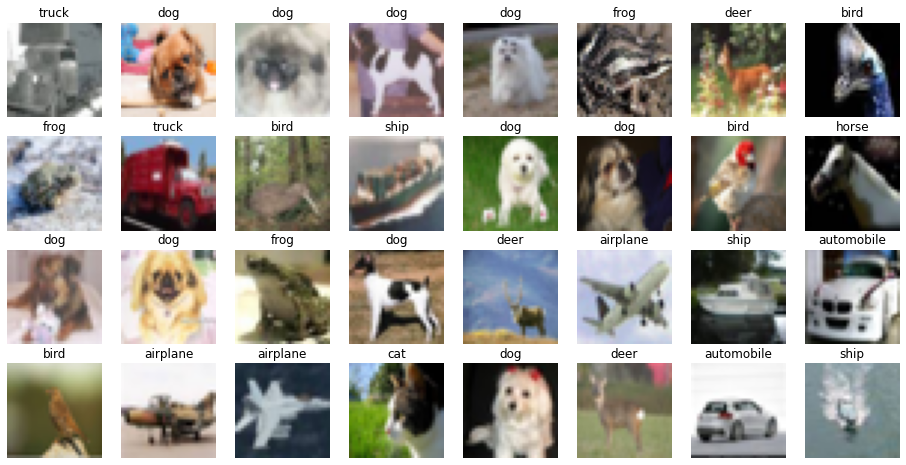## Prepare Training and Testing Data¶

Before defining the model and training the model, let us prepare the training and testing data.

In :
import tensorflow as tf
import numpy as np
print("TensorFlow's version is", tf.__version__)
print("Keras' version is", tf.keras.__version__)

TensorFlow's version is 2.2.0
Keras' version is 2.3.0-tf


Normalize the inputs, to train the model faster and prevent exploding gradients.

In :
# Normalize training and testing pixel values
X_train_normalized = X_train / 255 - 0.5
X_test_normalized = X_test / 255 - 0.5


Convert the labels to one-hot coded vectors.

In :
# Convert class vectors to binary class matrices.
Y_train_coded = tf.keras.utils.to_categorical(Y_train, NUM_CLASSES)
Y_test_coded = tf.keras.utils.to_categorical(Y_test, NUM_CLASSES)


## Define Convolutional Neural Network Model¶

Next, let us define a model that takes images as input, and outputs class probabilities.

We will define following layers in the model:

• Convolutional layer which takes (32, 32, 3) shaped images as input, outputs 16 filters, and has a kernel size of (3, 3), with the same padding, and uses LeakyReLU as activation function
• Convolutional layer which takes (32, 32, 16) shaped tensor as input, outputs 32 filters, and has a kernel size of (3, 3), with the same padding, and uses LeakyReLU as activation function
• Max Pool layer with pool size of (2, 2), this outputs (16, 16, 16) tensor
• Dropout layer with the dropout rate of 0.25, to prevent overfitting
• Convolutional layer which takes (16, 16, 16) shaped tensor as input, outputs 32 filters, and has a kernel size of (3, 3), with the same padding, and uses LeakyReLU as activation function
• Convolutional layer which takes (16, 16, 32) shaped tensor as input, outputs 64 filters, and has a kernel size of (3, 3), with the same padding, and uses LeakyReLU as activation function
• Max Pool layer with pool size of (2, 2), this outputs (8, 8, 64) tensor
• Dropout layer with the dropout rate of 0.25, to prevent overfitting
• Dense layer which takes input from 8x8x64 neurons, and has 256 neurons
• Dropout layer with the dropout rate of 0.5, to prevent overfitting
• Dense layer with 10 neurons, and softmax activation, is the final layer

As you can see, all the layers use LeakyReLU activations, except the last layer. This is a pretty good choice most of the time, but you change these as well to play with other activations such as tanh, sigmoid, ReLU, etc.

In :
# import necessary building blocks
from tensorflow.keras.models import Sequential
from tensorflow.keras.layers import Conv2D, MaxPooling2D, Flatten, Dense, Activation, Dropout
from tensorflow.keras.layers import LeakyReLU

def make_model():
"""
Returns Sequential model.
"""

model = Sequential()

return model

In :
# describe model
s = tf.keras.backend.clear_session()
model = make_model()
model.summary()

Model: "sequential"
_________________________________________________________________
Layer (type)                 Output Shape              Param #
=================================================================
conv2d (Conv2D)              (None, 32, 32, 16)        448
_________________________________________________________________
leaky_re_lu (LeakyReLU)      (None, 32, 32, 16)        0
_________________________________________________________________
conv2d_1 (Conv2D)            (None, 32, 32, 32)        4640
_________________________________________________________________
leaky_re_lu_1 (LeakyReLU)    (None, 32, 32, 32)        0
_________________________________________________________________
max_pooling2d (MaxPooling2D) (None, 16, 16, 32)        0
_________________________________________________________________
dropout (Dropout)            (None, 16, 16, 32)        0
_________________________________________________________________
conv2d_2 (Conv2D)            (None, 16, 16, 32)        9248
_________________________________________________________________
leaky_re_lu_2 (LeakyReLU)    (None, 16, 16, 32)        0
_________________________________________________________________
conv2d_3 (Conv2D)            (None, 16, 16, 64)        18496
_________________________________________________________________
leaky_re_lu_3 (LeakyReLU)    (None, 16, 16, 64)        0
_________________________________________________________________
max_pooling2d_1 (MaxPooling2 (None, 8, 8, 64)          0
_________________________________________________________________
dropout_1 (Dropout)          (None, 8, 8, 64)          0
_________________________________________________________________
flatten (Flatten)            (None, 4096)              0
_________________________________________________________________
dense (Dense)                (None, 256)               1048832
_________________________________________________________________
leaky_re_lu_4 (LeakyReLU)    (None, 256)               0
_________________________________________________________________
dropout_2 (Dropout)          (None, 256)               0
_________________________________________________________________
dense_1 (Dense)              (None, 10)                2570
_________________________________________________________________
activation (Activation)      (None, 10)                0
=================================================================
Total params: 1,084,234
Trainable params: 1,084,234
Non-trainable params: 0
_________________________________________________________________


Next, we train the model that we defined above. We will use 0.005 as our initial learning rate, training batch size will be 64, we will train our model for 10 epochs. Feel free to change these hyperparameters, to dive deeper and know their effects. We use categorical cross entropy loss as our lass function and Adamax optimizer for convergence.

In :
INIT_LR = 5e-3  # initial learning rate
BATCH_SIZE = 64
EPOCHS = 10

s = tf.keras.backend.clear_session()  # clear default graph
# don't call K.set_learning_phase() !!! (otherwise will enable dropout in train/test simultaneously)
model = make_model()  # define our model

# prepare model for fitting (loss, optimizer, etc)
model.compile(
loss='categorical_crossentropy',  # we train 10-way classification
metrics=['accuracy']  # report accuracy during training
)


We define a learning rate scheduler, which decays learning rate after each epoch.

In :
# scheduler of learning rate (decay with epochs)
def lr_scheduler(epoch):
return INIT_LR * 0.9 ** epoch


We also define a class that handles callbacks from keras. It prints out the learning rate used in that epoch.

In :
# callback for printing of actual learning rate used by optimizer
class LrHistory(tf.keras.callbacks.Callback):
def on_epoch_begin(self, epoch, logs={}):
print("Learning rate:", tf.keras.backend.get_value(model.optimizer.lr))


Now, let us train our model on normalized X_train, X_train_normalized, and one-hot coded matrix, Y_train_coded. During training we will also keep validating on, X_test_normalized and Y_train_coded. In this way we can keep an eye on model performance.

In :
# fit model
history = model.fit(
X_train_normalized, Y_train_coded,  # prepared data
batch_size=BATCH_SIZE,
epochs=EPOCHS,
callbacks=[tf.keras.callbacks.LearningRateScheduler(lr_scheduler),
LrHistory()],
validation_data=(X_test_normalized, Y_test_coded),
shuffle=True,
verbose=1,
initial_epoch=0
)

Learning rate: 0.005
Epoch 1/10
782/782 [==============================] - 67s 85ms/step - loss: 1.3914 - accuracy: 0.5013 - val_loss: 1.0452 - val_accuracy: 0.6284 - lr: 0.0050
Learning rate: 0.0045
Epoch 2/10
782/782 [==============================] - 68s 87ms/step - loss: 0.9876 - accuracy: 0.6523 - val_loss: 0.8390 - val_accuracy: 0.7021 - lr: 0.0045
Learning rate: 0.00405
Epoch 3/10
782/782 [==============================] - 67s 85ms/step - loss: 0.8475 - accuracy: 0.7028 - val_loss: 0.7810 - val_accuracy: 0.7250 - lr: 0.0041
Learning rate: 0.003645
Epoch 4/10
782/782 [==============================] - 67s 85ms/step - loss: 0.7470 - accuracy: 0.7357 - val_loss: 0.7199 - val_accuracy: 0.7471 - lr: 0.0036
Learning rate: 0.0032805
Epoch 5/10
782/782 [==============================] - 77s 98ms/step - loss: 0.6782 - accuracy: 0.7637 - val_loss: 0.6910 - val_accuracy: 0.7628 - lr: 0.0033
Learning rate: 0.00295245
Epoch 6/10
782/782 [==============================] - 97s 124ms/step - loss: 0.6315 - accuracy: 0.7783 - val_loss: 0.6735 - val_accuracy: 0.7681 - lr: 0.0030
Learning rate: 0.002657205
Epoch 7/10
782/782 [==============================] - 97s 124ms/step - loss: 0.5906 - accuracy: 0.7931 - val_loss: 0.6372 - val_accuracy: 0.7773 - lr: 0.0027
Learning rate: 0.0023914846
Epoch 8/10
782/782 [==============================] - 97s 124ms/step - loss: 0.5558 - accuracy: 0.8035 - val_loss: 0.6367 - val_accuracy: 0.7813 - lr: 0.0024
Learning rate: 0.002152336
Epoch 9/10
782/782 [==============================] - 97s 124ms/step - loss: 0.5288 - accuracy: 0.8121 - val_loss: 0.6323 - val_accuracy: 0.7858 - lr: 0.0022
Learning rate: 0.0019371024
Epoch 10/10
782/782 [==============================] - 88s 113ms/step - loss: 0.4963 - accuracy: 0.8229 - val_loss: 0.6135 - val_accuracy: 0.7911 - lr: 0.0019

In :
def save_model(model):# serialize model to JSON
model_json = model.to_json()
with open("model.json", "w") as json_file:
json_file.write(model_json)
# serialize weights to HDF5
model.save_weights("model.h5")
print("Saved model to disk")

save_model(model)

Saved model to disk


## Evaluate the model¶

Now that we have trained our model, let us see how it performs.

Let us load the saved model from disk.

In :
def load_model():
from tensorflow.keras.models import model_from_json

# load json and create model
json_file = open('model.json', 'r')
json_file.close()
# load weights into new model


Loaded model from disk


Let us look at the learning curve during the training of our model.

In :
plt.plot(history.history['loss'])
plt.plot(history.history['val_loss'])
plt.title('model loss')
plt.ylabel('loss')
plt.xlabel('epoch')
plt.legend(['train', 'test'], loc='upper left')
plt.show()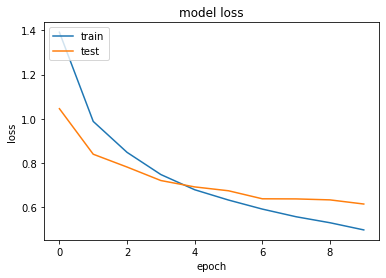Let us predict the classes for each image in testing set.

In :
# make test predictions
Y_pred_test = model.predict_proba(X_test_normalized) # Predict probability of image belonging to a class, for each class
Y_pred_test_classes = np.argmax(Y_pred_test, axis=1) # Class with highest probability from predicted probabilities
Y_test_classes = np.argmax(Y_test_coded, axis=1) # Actual class
Y_pred_test_max_probas = np.max(Y_pred_test, axis=1) # Highest probability

WARNING:tensorflow:From <ipython-input-17-1f2ff8baac59>:2: Sequential.predict_proba (from tensorflow.python.keras.engine.sequential) is deprecated and will be removed after 2021-01-01.
Instructions for updating:
Please use model.predict() instead.


Let us look at the confusion matrix to understand the performance of our model.

In :
# confusion matrix and accuracy
from sklearn.metrics import confusion_matrix, accuracy_score
plt.figure(figsize=(7, 6))
plt.title('Confusion matrix', fontsize=16)
plt.imshow(confusion_matrix(Y_test_classes, Y_pred_test_classes))
plt.xticks(np.arange(10), CIFAR10_CLASSES, rotation=45, fontsize=12)
plt.yticks(np.arange(10), CIFAR10_CLASSES, fontsize=12)
plt.colorbar()
plt.show()
print("Test accuracy:", accuracy_score(Y_test_classes, Y_pred_test_classes))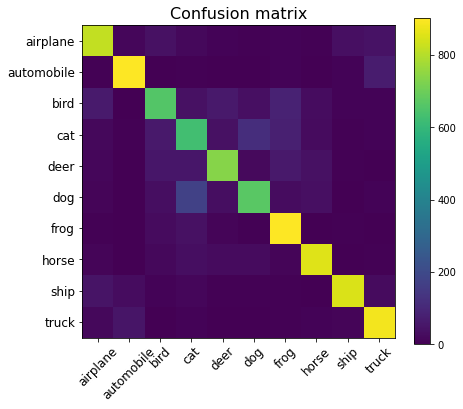Test accuracy: 0.7911


Let us look at some random predictions from our model.

In :
# inspect preditions
cols = 8
rows = 2
fig = plt.figure(figsize=(2 * cols - 1, 3 * rows - 1))
for i in range(cols):
for j in range(rows):
random_index = np.random.randint(0, len(Y_test))
ax = fig.add_subplot(rows, cols, i * rows + j + 1)
ax.grid(b=False)
ax.axis('off')
ax.imshow(X_test[random_index, :])
pred_label = CIFAR10_CLASSES[Y_pred_test_classes[random_index]]
pred_proba = Y_pred_test_max_probas[random_index]
true_label = CIFAR10_CLASSES[Y_test[random_index]]
ax.set_title("pred: {}\nscore: {:.3}\ntrue: {}".format(
pred_label, pred_proba, true_label
))
plt.show()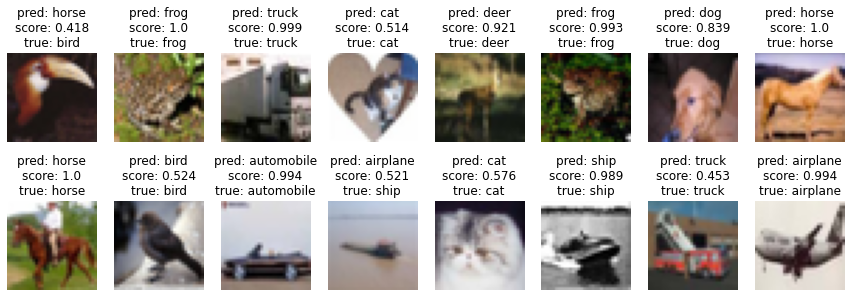## Summary¶

In this tutorial, we discovered how to develop a convolutional neural network for CIFAR-10 classification from scratch using TensorFlow.

Specifically, we learned:

• How to look at random images in the dataset
• How to define and train a model
• How to save the learnt weights of the model to disk
• How to predict clsses using the model

These topics will be covered later:

• How to improve your model
• How to thoroughly validate your model

This is a pretty good model (if it is among your first few), but people have achieved around 99% accuracy in this dataset. You can checkout other people's performance on this dataset here.

If you want to work on this model on your system, you can find the code here.

In :
saved_model_dir = 'cifar-10_model.TF'
model.save(saved_model_dir)

WARNING:tensorflow:From /opt/tljh/user/lib/python3.7/site-packages/tensorflow/python/ops/resource_variable_ops.py:1817: calling BaseResourceVariable.__init__ (from tensorflow.python.ops.resource_variable_ops) with constraint is deprecated and will be removed in a future version.
Instructions for updating:
If using Keras pass *_constraint arguments to layers.
INFO:tensorflow:Assets written to: cifar-10_model.TF/assets

In :
tflite_model_file = "cifar-10_converted_model.tflite"
converter = tf.lite.TFLiteConverter.from_saved_model(saved_model_dir)
tflite_model = converter.convert()
open(tflite_model_file, "wb").write(tflite_model)

Out:
4343500
In :
tflite_quantized_model_file = "converted_quantized_model.tflite"
converter.optimizations = [tf.lite.Optimize.DEFAULT]
tflite_quantized_model = converter.convert()
open(tflite_quantized_model_file, "wb").write(tflite_quantized_model)

Out:
1093856
In :
!deepCC cifar-10_model.TF --format=tensorflow

reading [tensorflow model] from 'cifar-10_model.TF'
Saved 'cifar-10_model.TF.onnx'
reading onnx model from file  cifar-10_model.TF.onnx
Model info:
ir_vesion :  4
doc       :
WARN (ONNX): terminal (input/output) conv2d_input_0's shape is less than 1.
changing it to 1.
WARN (ONNX): terminal (input/output) Identity_0's shape is less than 1.
changing it to 1.
running DNNC graph sanity check ... passed.
Writing C++ file  cifar-10_model.TF_deepC/cifar-10_model.TF.cpp
INFO (ONNX): model files are ready in dir cifar-10_model.TF_deepC
g++ -O3 -I. -I/opt/tljh/user/lib/python3.7/site-packages/deepC-0.13-py3.7-linux-x86_64.egg/deepC/include -isystem /opt/tljh/user/lib/python3.7/site-packages/deepC-0.13-py3.7-linux-x86_64.egg/deepC/packages/eigen-eigen-323c052e1731 cifar-10_model.TF_deepC/cifar-10_model.TF.cpp -o cifar-10_model.TF_deepC/cifar-10_model.TF.exe
Model executable  cifar-10_model.TF_deepC/cifar-10_model.TF.exe


## Display an image¶

In :
from PIL import Image
import matplotlib.pyplot as plt

img_data = X_test
np.savetxt('img.data', img_data.flatten())

img = Image.fromarray(img_data, 'RGB')
#print(img)
img.show()
plt.imshow(img_data)

Out:
<matplotlib.image.AxesImage at 0x7f476cfcd4e0>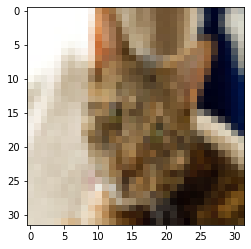## Run prediction on the image shown above¶

In :
!./cifar-10_model.TF_deepC/cifar-10_model.TF.exe img.data

reading file img.data.
writing file Identity_0.out.

In :
nn_out = np.loadtxt('Identity_0.out')
print ("Real label : ", CIFAR10_CLASSES[int(Y_test)])
print ("Keras Prediction : ", CIFAR10_CLASSES[np.argmax(model.predict(X_test))])
print ("DeepC prediction is : ", CIFAR10_CLASSES[np.argmax(nn_out)])

Real label :  cat
Keras Prediction :  truck
DeepC prediction is :  truck